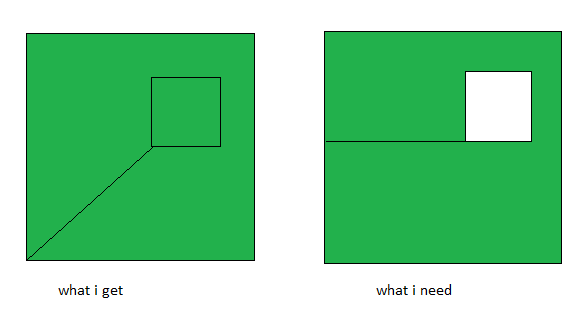# get different extra edge for hollow polygon

• the following method returns points:

``````QPolygonF(QPointF(1,1) QPointF(1,6) QPointF(6,6) QPointF(6,1) QPointF(1,1) QPointF(2,2) QPointF(2,3) QPointF(3,3) QPointF(3,2) QPointF(2,2) QPointF(1,1))
``````
``````#include <QPolygonF>
#include <QDebug>

int main()
{
QPolygonF inner;
inner.push_back({ 2, 2 });
inner.push_back({ 2, 3 });
inner.push_back({ 3, 3 });
inner.push_back({ 3, 2 });

QPolygonF outer;
outer.push_back({ 1, 1 });
outer.push_back({ 1, 6 });
outer.push_back({ 6, 6 });
outer.push_back({ 6, 1 });

const auto &hollowPoly = outer.subtracted(inner);
qDebug() << hollowPoly;
}
``````

what i need is to have the following points:

``````QPolygonF(QPointF(1,1) QPointF(1,6) QPointF(6,6) QPointF(6,1) QPointF(1,1) QPointF(1,2) QPointF(2,2) QPointF(3,2) QPointF(3,3) QPointF(2,3) QPointF(2,2) QPointF(1,2))
``````

is that possible to get?

• is that possible to get?

Why not? Did you try?

• @jsulm
how? is there method that will return such result?

• @user4592357 Can you please explain better what you want to do? Because it is not clear to me...

• @jsulm
from docs:

``````QPolygonF QPolygonF::subtracted(const QPolygonF &r) const
Set operations on polygons will treat the polygons as areas.
``````

due to this the coordinates of the resulting polygon aren't as expected by renderer, so it doesn't draw the hollow part but instead fills it will color.
i need to get the other set of points as resulting polygon so that the renderer will draw the hollow part. in that set of points, outer vertices are in clockwise, and inner vertices in counter-clockwise direction.

• Hi,

I think I follow your query but could you provide an image of the result you are expecting ?

• @SGaist
sure.• @SGaist do you have a suggestion how to do this?

• Which version of Qt are you using ?
Can you complete your example so that we also have the same drawing output ?